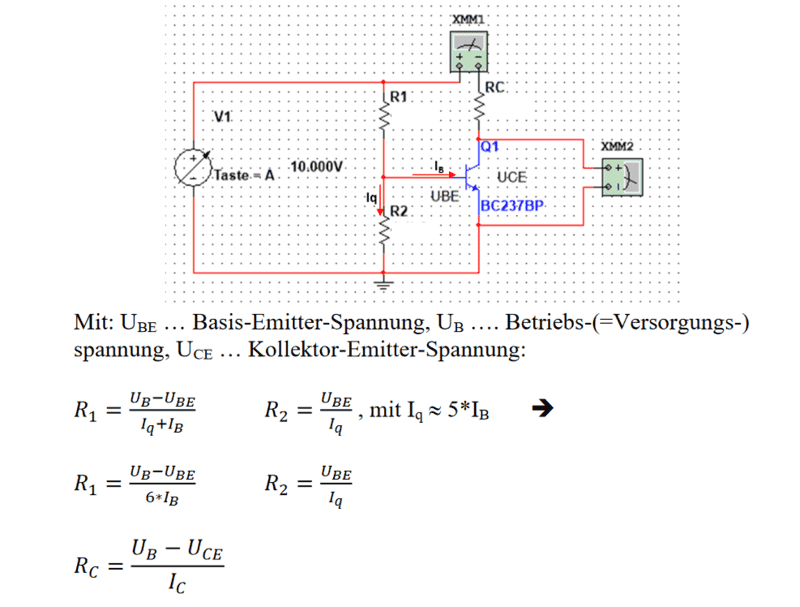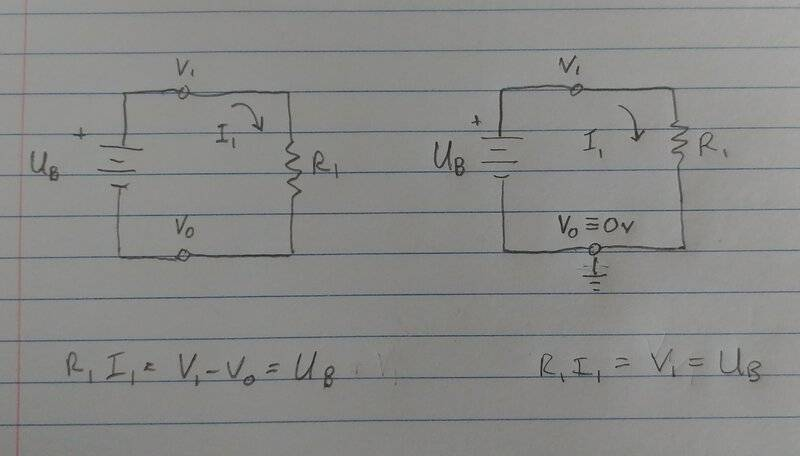# BJT emitter circuit weird equations?

• altruan23
In summary, the equations shown in the conversation are correct. The confusion seems to stem from the different ways that voltage is expressed and the importance of defining a common reference point, or "ground," in circuit analysis. It is recommended to define and use clear and consistent notation to avoid confusion. It is also common practice to redraw and rename elements in a circuit to match preferred definitions.f

#### altruan23

Summary:: So apparently the equations schould be correct but I am not sure about it.Isnt R1 = Ub(10V) - Base voltage / Iq + Ib --> Ub is the source voltage 10V.
And schouldnt R2 be :base voltage / Iq?

Too many definitions. V1 UB ?

•DaveE
V1 is 10 V and that is Ub. Our teacher used the name Ub as source voltage, altought usually Ub is meaning base voltage but not in this example.

•DaveE
Summary:: So apparently the equations schould be correct but I am not sure about it.

View attachment 297780

Isnt R1 = Ub(10V) - Base voltage / Iq + Ib --> Ub is the source voltage 10V.
And schouldnt R2 be :base voltage / Iq?
I don't understand. Your questions are exactly* what those equations say. I think your questions aren't so much about the physics of voltage drops across resistors and Ohm's law as they are about dealing with sloppy definitions. Define the voltages and currents carefully and try to be consistent in their use.

The equations shown are correct.

* Parentheses matter. When you write "Ub(10V) - Base voltage / Iq + Ib" This means ##U_B-\frac{U_{BE}}{I_q}+I_B## . I don't think that's what you meant.

•hutchphd
I don't understand how can we use Ube if this voltage is between the terminals B and E from transistor.
Arent we allowed to use only the voltage from Terminal B to ground? that means base voltage?

Perhaps your concern relates to the different ways that voltage is expressed in the practice of circuit analysis. Voltage is really only a useful concept as a voltage difference (i.e. the voltage across a resistor). In a circuit example that includes finding the current in a resistor, there is no real difference in that current between a voltage drop from 1002V to 1001V as from 2V to 1V or 1V to 0V. However, to make it easier for us to write and solve equations, we will often use a single voltage which is implicitly referenced to a very specific single voltage in the circuit. We would normally call this voltage "ground" and define its value to be zero.

So, The simple example I drew below shows how these representations are equivalent, provided you understand that the "ground" voltage ##V_0## has been defined to be zero and thus left out of the equations.Also, once the ground voltage has been define to be zero, that applies to all of the circuit elements connected to that node. So this is the same as saying the emitter voltage in your circuit is defined to be zero and thus ##U_{BE}## could also be referred to as the base voltage (with respect to ground).

As a matter of style, to avoid confusion, I really dislike mixing the variables named ##U## and ##V## to express voltages. It is much easier to read the equations if there is a common standard notation.

Finally, I will add that in many decades of working with circuits like this, it has been really common for me to simply redraw and rename things in the circuit to match my preferred definitions. Otherwise things can get unnecessarily confusing.

•altruan23
thank you very much for the long answer! very nice from you! it is clearer now! thanks again!

•DaveE